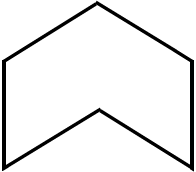# Area

## Objective

Understand that area is an attribute of plane figures that is measured in square units. Find the area of a figure using pattern blocks, which can be used as concrete non-standard units.

## Common Core Standards

### Core Standards

?

• 3.MD.C.5 — Recognize area as an attribute of plane figures and understand concepts of area measurement.

• 3.MD.C.6 — Measure areas by counting unit squares (square cm, square m, square in, square ft, and improvised units).

?

• 1.G.A.2

## Criteria for Success

?

1. Understand that area is the measure of how much flat space an object takes up.
2. Compare the areas of various figures that have some common dimension or property.
3. Find the area of various figures by covering a space with concrete non-standard units without gaps or overlaps (MP.5, MP.6).
4. Find the area of a figure multiple times, using units of different sizes for the different measurements.
5. Explain how multiple measurements of the same figure is related to the size of the unit chosen (MP.3).

## Tips for Teachers

?

• Lesson 1 begins a progression throughout all of Topic A in which students “determine the number of squares in each row with increasingly sophisticated strategies, such as skip-counting the number in each row and eventually multiplying the number in each row by the number of rows (MP.8)” (GM Progression, p. 17).
• The Problem Set mirrors the Anchor Tasks quite closely, but with pattern blocks as a non-standard unit to measure area instead of the tangram set. You might decide to just use the Anchor Tasks as an exploration for the whole period or skip the Anchor Tasks and make the Problem Set a more guided activity. Both are included here, however, so that students can do some guided exploration as well as independent practice of measuring area with concrete non-standard units, if you so choose.

### Lesson Materials

• Template: Tangrams (1 per student) — This material should be cut into pieces before the lesson.
• Pattern blocks (6 triangles, 3 blue (fatter) rhombuses, 2 trapezoids per student or small group) — These are needed for the Problem Set.

### Remote Learning Guidance

If you need to adapt or shorten this lesson for remote learning, we suggest prioritizing Anchor Task 2 (benefits from discussion) and Anchor Task 3 (benefits from worked example). Find more guidance on adapting our math curriculum for remote learning here.

#### Fishtank Plus

• Problem Set
• Student Handout Editor
• Vocabulary Package

?

### Problem 1

Does the large triangle or square take up more space? Justify your answer.

### Problem 2

Area is the measure of how much flat space an object takes up. Compare the areas of the medium triangle and the parallelogram.

### Problem 3

Use copies of the smallest triangle to determine the area of each of the shapes.

## Problem Set & Homework

### Discussion of Problem Set

• How many triangle pattern blocks does it take to cover a rhombus pattern block? Use that information to say a division fact that relates the number of triangles it takes to cover Shape A to the number of rhombuses it takes to cover the same shape. ($6\div 2 = 3$
• What new math vocabulary did we use today to communicate precisely about the amount of space taken up by a shape?

?

Use triangle pattern blocks to find the area of the shape below.Area: _____ triangle units